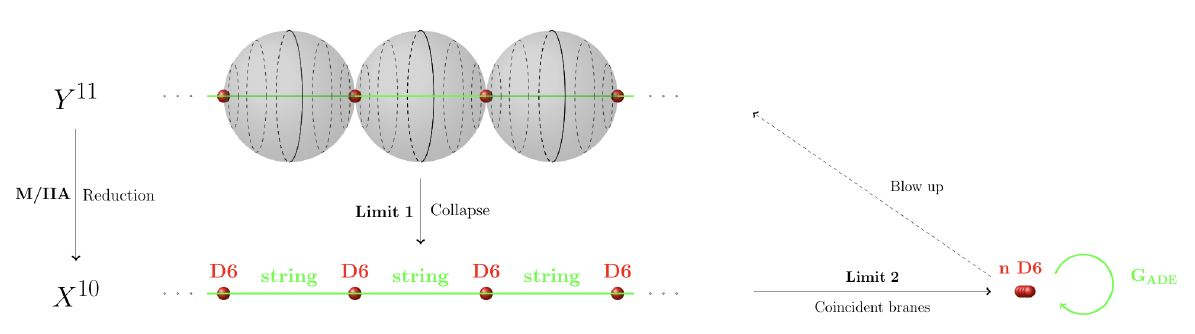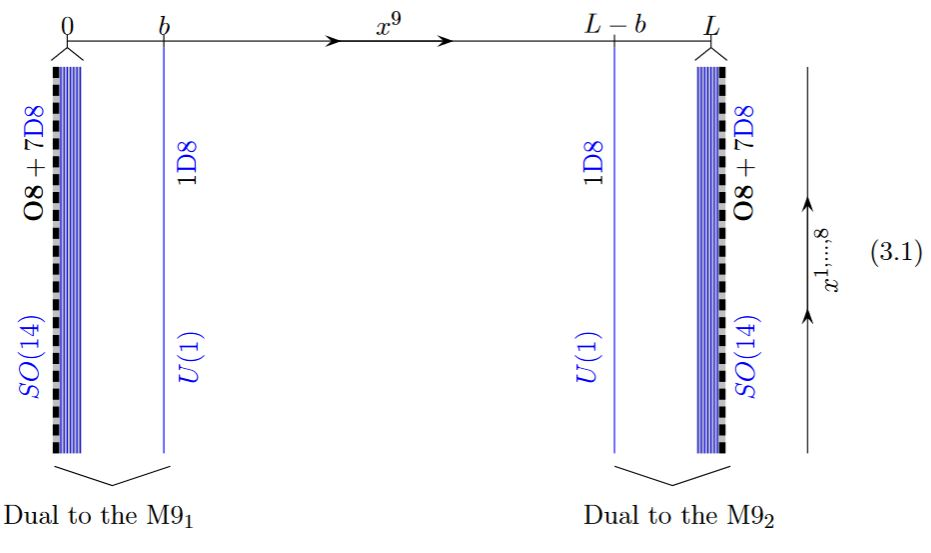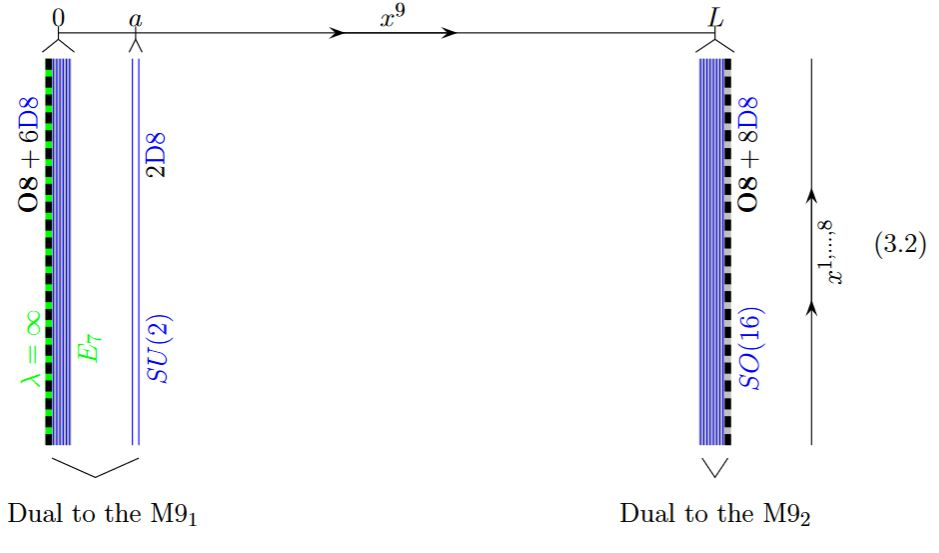# nLab enhanced gauge symmetry

Contents

## Surveys, textbooks and lecture notes

#### Chern-Weil theory

∞-Chern-Weil theory

∞-Chern-Simons theory

∞-Wess-Zumino-Witten theory

# Contents

## Idea

Generally, an enhanced gauge symmetry refers to the appearance of gauge theory with a larger gauge group than may be superficially apparent.

### On coincident D-branes

A famous case is the enhanced gauge symmetry in the Chan-Paton gauge fields of coincident D-branes. A priori each D-brane carries a complex line bundle/circle principal bundle, but as $n$ of them coincide the gauge group is expected to enhance from $(U(1))^n$ to the unitary group $U(n)$ (or the special unitary group $SU(n)$).

Mathematically this gauge enhancement on D-branes is modeled by the Baum-Douglas geometric cycle model for K-homology (Reis-Szabo 05, def. 1.5 and p. 16, Szabo 08, p. 4): One such cycle is given by a submanifold $W \overset{\phi}{\hookrightarrow} X$ of spacetime $X$ equipped with spin^c structure and with a complex vector bundle $E \to X$, and given two such cycles $(W,\phi,E_1)$ and $(W,\phi,E_2)$ with the same underlying manifold (“coincident D-brane worldvolume”) then their formal direct sum is identified with the single cycle carrying the direct sum of vector bundles

$(W,\phi, E_1) + (W, \phi, E_2) \;\sim\; (W, \phi, E_1 \oplus E_2) \,.$

If here $E_i$ has rank $n_i$ and hence structure group $U(n_i)$, then the direct sum of vector bundles $E_1 \oplus E_2$ has structure group the product group $U(n_1) \times U(n_2)$.

In particular the sum of $n$ coincident cycles each carrying a line bundle yields a rank-$n$ vector bundle with structure group $(U(1))^n$. In this sense then a cycle carrying a general rank $n$-vector bundle, hence with structure group $U(n)$, may be thought of as a “gauge enhancement” of making $n$ D-branes coincide.

### From massless BPS states

In the context of the realization of super Yang-Mills theories as effective field theories of superstring theory, BPS branes in the string background becomes BPS states of the SYM theory. These become massless as the cycles on which the BPS charge is supported shrink away (at boundary points of the given moduli space). In this case these additional massless states may appear as additional gauge bosons in the compactified gauge theory which thereby develops a larger gauge group. Typically a previously abelian gauge group becomes non-abelian this way.

Examples include KK-compactification of M-theory on K3 fibers and on G2-manifolds with ADE singularities, corresponding to F-theory on elliptic fibrations making K3-fibrations with singular fibers, corresponding to heterotic string theory on singular elliptic fibrations. See at

### For MK6 branes at ADE-singularities

The blow-up of an ADE-singularity is given by a union of Riemann spheres that touch each other such as to form the shape of the Dynkin diagram whose A-D-E label corresponds to that of the given finite subgroup of SU(2).

This statement is originally due to (duVal 1934 I, p. 1-3 (453-455)). A description in terms of hyper-Kähler geometry is due to Kronheimer 89a.

Quick survey of this fact is in Reid 87, a textbook account is Slodowy 80.

In string theory this fact is interpreted in terms of gauge enhancement of the M-theory-lift of coincident black D6-branes to an MK6 at an ADE-singularity (Sen 97):graphics grabbed from HSS18

See at M-theory lift of gauge enhancement on D6-branes for more.

### On M9-Branes

In Horava-Witten theory there is supposed to be gauge enhancement over the M9-branes such as to identify their worldvolume theory with E8-heterotic string theory.

Under the duality between M-theory and type IIA string theory the M9-brane may be identified with the O8-plane:This may be used to understand the gauge enhancement to E8-gauge groups at the heterotic boundary of Horava-Witten theory:### On coincident D-branes

The idea that on $N$ coincident D-branes there is gauge enhancement to $U(N)$-gauge field theory is due to

There, this is called an “obvious guess” (first line on p. 8). Subsequently, most authors cite this obvious guess as a fact; for instance the review

Discussion in terms of boundary conformal field theory is in

By actual computation in open string field theory “convincing evidence” for the nonabelian Yang-Mills theory on coincident D-branes was found, numerically, in (see bottom of p. 22)

Similar numerical derivation, as well as exact derivation at zero momentum, is in

The first full derivation seems to be due to

• Taejin Lee, Covariant open bosonic string field theory on multiple D-branes in the proper-time gauge, Journal of the Korean Physical Society December 2017, Volume 71, Issue 12, pp 886–903 (arXiv:1609.01473)

which is surveyed in

That on D0-branes this reproduces the BFSS matrix model and on D(-1)-branes the IKKT matrix model is shown in

• Taejin Lee, Covariant Open String Field Theory on Multiple D$p$-Branes (arXiv:1703.06402)

Discussion of gauge enhancement on coincident D-branes in terms of Baum-Douglas geometric cycles for K-homology is in

• Rui Reis, Richard Szabo, Geometric K-Homology of Flat D-Branes ,Commun.Math.Phys. 266 (2006) 71-122, Journal of the Korean Physical Society December 2017, Volume 71, Issue 12, pp 886–903 (arXiv:hep-th/0507043)

reviewed in

Derivation via rational parameterized stable homotopy theory applied to The brane bouquet is in

The picture of M-theory lift of gauge enhancement on D6-branes is due to

Review of the standard lore of gauge enhancement in M-theory includes

(…)

### General

Original articles include

Discussion via duality of M9-branes to O-planes is in

• E. Gorbatov, V.S. Kaplunovsky, J. Sonnenschein, Stefan Theisen, S. Yankielowicz, section 3 of On Heterotic Orbifolds, M Theory and Type I’ Brane Engineering, JHEP 0205:015, 2002 (arXiv:hep-th/0108135)